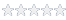# How to calculate the frequency measurement accuracy of the GTX22x0 series of…

#### Knowledge Base Article # Q200171

Summary How to calculate the frequency measurement accuracy for the GTX22x0 seriesLogin to rate article

Many factors can effect the accuracy of frequency measurements taken on any instrument.

Main factors that effect accuracy are:

1) Signal noise
2) Instrument timebase accuracy
3) Gate time used to measure the signal
4) Calibration cycle

 GTX2200 SeriesLearn More14 measurement functionsTime Interval Counter PXI Card

The frequency measurement accuracy for the GTX2200 series can be found using the following formulas:

Slew Rate
Slew Rate (SR) = 2 x PI x Vp x f
where PI = 3.14159265
Vp = peak amplitude of the sine wave being measured (in volts)
f = frequency of the signal being measured

Trigger Error
Error due to noise superimposed on the input signal from both internal and external sourcesEn = rms noise of input signal (100MHz bandwidth)

Least Significant Digit (LSD)Resolution (Hertz)Accuracy (Hertz)The following Excel file can be used to calculate the accuracy GTX22x0 Accuracy Spreadsheet .The values for Signal Frequency, Signal Noise (En), Measurement Gate Time and Instrument Timebase accuracy can be adjusted to observe the effects of these parameters on the overall measurement accuracy.
Article Date 7/10/2009 , 9/21/2021
Keywords GTX2200, GTX2210, GTX2220, GTX2230, accuracy

 Login to rate article>